# Trigonometric Identities## Trigonometric Identities

A mathematical statement of equality involving the trigonometrical ratios with one or more variable angle and is true for any value of the angle is called a trigonometric identity or trig identity.

********************

10 Math Problems officially announces the release of Quick Math Solver, an Android App on the Google Play Store for students around the world.

********************

Generally, α, β, θ, or A, B, C symbols are used for the variable angle in the trigonometric identities.Here are some examples of trigonometric identities:etc.

### Standard Trigonometric Identities (Trig identities)

Here are some standard trigonometric identities (trig identities) considered as the trigonometric formulas as well:### Derived Trigonometric Relations:

We can derive some other trigonometric relations from the standard trigonometic identities. Here are those derived trigonometric relations:## How to Prove Trigonometric Identities?

To prove a trigonometric identity, we have to show that the expressions on both the sides of equality sign are equal. For that, we take left hand side (LHS) and simplify it to the expression equal to the right hand side (RHS) or vice-versa.

We simplify the trigonometric expression by a process similar to algebraic simplification. We can use almost all the algebraic formulas, methods of factorization, rational simplification, division, multiplication, addition, and subtraction.

Moreover, we use here the standard trigonometric identities and the derived relations as the formula while simplifying the trigonometric expressions.

For example:

Prove: (sinθ + cosθ)2 = 1 + 2sinθcosθ

Solution: Here,

LHS = (sinθ + cosθ)2

= sin2θ + 2sinθcosθ + cos2θ [ (a + b)2 = a2 + 2ab + b2]

= sin2θ + cos2θ + 2sinθcosθ

= 1 + 2sinθcosθ [ sin2θ + cos2θ = 1]

= RHS. Proved.

### Rules for proving trigonometric identities

1.     Take LHS and simplify to get RHS, if LHS is complicated.

2.     Take RHS and simplify to get LHS, if RHS is complicated.

3.     If LHS and RHS both are complicated, take LHS and RHS both and simplify them to a common result.

4.     If the given identity is more complicated then change it to a simpler form by transposing or applying the method of cross-multiplication. Then, prove the new identity by the above procedure.

### List of Trigonometric Formulas (Trig Formulas)## Proving Trigonometric Identities

### Worked out Examples:

Example 1: Prove that: cosecA.tanA.cosA = 1

Solution: Here,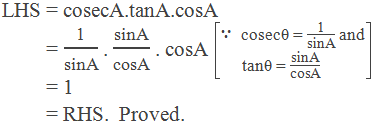Example 2: Prove that: sec2θ (1 – cos2θ) =  tan2θ

Solution: Here,Example 3: Prove that: sin2θ . tan2θ = tan2θ – sin2θ

Solution: Here,Example 4: Prove that: tan4A + tan2A = sec4A – sec2A

Solution: Here,

LHS = tan4A + tan2A

= tan2A (tan2A + 1)

= (sec2A – 1) sec2A

= sec4A – sec2A

= RHS.  Proved.

Example 5: Prove that:Solution: Here,Example 6: Prove that: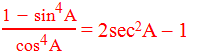Solution: Here,Example 7: Prove that: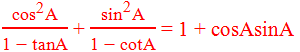Solution: Here,Example 8: Prove that: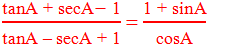Solution: Here,Example 9: Prove that: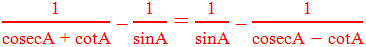Solution: Here,Example 10: Prove that: (3 – 4sin2A)(sec2A – 4tan2A) = (3 – tan2A)(1 – 4sin2A)

Solution: Here,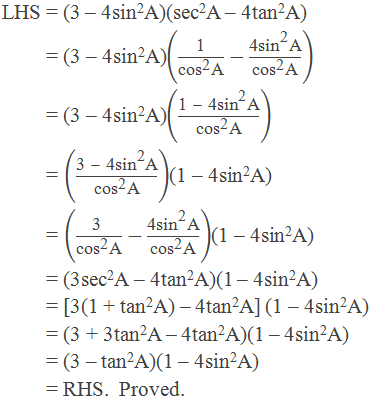#### Do you have any questions or problems regarding the Trigonometric Identities?

You can ask here, in the comment section below.